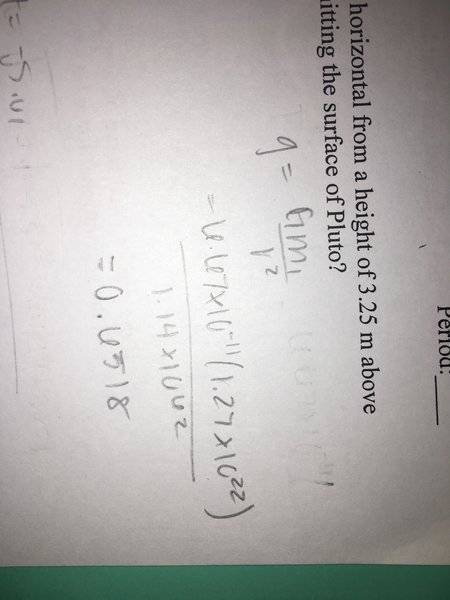# Projectile problem on Pluto

## Homework Statement

An astronaut throws a rock at 12 m/s at an angle of 25° above horizontal from a height of 3.25 m above the surface. How far horizontally does the rock travel before hitting the surface of Pluto?

Pluto has a mass of 1.27 E22 kg
And a radius of 1.14E6 m

## Homework Equations

(Projectile equations )
d=vo(t) + .5a(t^2)
Dx=vx (t)

## The Attempt at a Solution

I know how to solve projectiles , except how do I find gravitational acceleration for Pluto ? [/B]

haruspex
Homework Helper
Gold Member
2020 Award
how do I find gravitational acceleration for Pluto ?
Do you know a formula involving the gravitational constant G?

Do you know a formula involving the gravitational constant G?

Fg = (Gm)/r^2 ?

haruspex
Homework Helper
Gold Member
2020 Award
Fg = (Gm)/r^2 ?
Not quite. If you have a force on the left then you need two masses on the right. Or you can have an acceleration on the left and just the attracting mass on the right.

Not quite. If you have a force on the left then you need two masses on the right. Or you can have an acceleration on the left and just the attracting mass on the right.

Fg=(G(m1)(m2))/r2
But then , where would the other mass come from ? Only one mass is given

haruspex
Homework Helper
Gold Member
2020 Award
Fg=(G(m1)(m2))/r2
But then , where would the other mass come from ? Only one mass is given
You only need to know the vertical acceleration of the rock. According to the formula above, the force is proportional to its mass, so the acceleration is independent of its mass.

You only need to know the vertical acceleration of the rock. According to the formula above, the force is proportional to its mass, so the acceleration is independent of its mass.

I don’t understand

haruspex
Homework Helper
Gold Member
2020 Award
I don’t understand
Let the mass of the rock be m. What is the gravitational force on it?

Let the mass of the rock be m. What is the gravitational force on it?

That’s what I’m trying to figure out

haruspex
Homework Helper
Gold Member
2020 Award
That’s what I’m trying to figure out
Use the formula you quoted in post #3. The unknown mass of the rock, m, appears in the answer.

Use the formula you quoted in post #3. The unknown mass of the rock, m, appears in the answer.

I’m not trying to find mass, the mass is given .
I’m looking for gravitational acceleration,
I keep getting 0.65 but I’m not sure if that’s correct

haruspex
Homework Helper
Gold Member
2020 Award
I’m not trying to find mass, the mass is given .
No, the mass you are given is the mass of the planet.
In post #5 you complained that you are not given the mass of the rock, so you cannot use the formula to find the force on it. I keep trying to explain to you that that is not a problem. You can find an expression for the force which includes the mass of the rock as an unknown.
I keep getting 0.65 but I’m not sure if that’s correct
0.65 what? m/s2?

No, the mass you are given is the mass of the planet.
In post #5 you complained that you are not given the mass of the rock, so you cannot use the formula to find the force on it. I keep trying to explain to you that that is not a problem. You can find an expression for the force which includes the mass of the rock as an unknown.

0.65 what? m/s2?haruspex